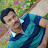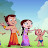IB MYP,HL,SL,AP Maths Tutor in Vancouver,Algebra,Geometry,Trigonometry,Calculus Skype:ykreddy22IB MYP,HL,SL,AP Maths Tutor in Vancouver,Algebra,Geometry,Trigonometry,Calculus Skype:ykreddy22

I am a full-time mathematics tutor offering a personalized Online Tutoring service for IB(International Baccalaureate),PYP, MYP and IB Diploma HL,SL
I am IB Math Coordinator and Examiner .I have in-depth knowledge of mathematics.How to get basics and concepts across to students.
I offer online tuition using Skype for IB Math HL, Math SL, Math studies or for AP level Math or A/AS levels Math or for IGCSE Math Core, extended
Expert Experience and Eminent Math tutor. This is kondall reddy (M sc Math Ed ) with 16 years of experience and expertise as math teacher or math tutor or
online math tutor for IGCSE,IB Students classes 6th to 12th .I believe that Every child is unique and every student have their own potentiality and creativity.
I teach to the students with basics and concepts from the beginning each topic.I feel that “Practice makes a Math perfect”
I can assure to make a difference in your Childs progress.
IBDP Math HL Past Papers,IB SL Math Past papers,IB Studies Level Past Papers from 2000 to 2015 all years papers help through online Skype: ykreddy22

algebra tutors,Online GCSE Maths Tutors & GCSE Maths Tuition,Private Maths Tuition,Maths Tutors North West London,IB MYP,HL,SL,AP Maths Tutor in Vancouver,Algebra,Geometry,Trigonometry,Calculus Skype:ykreddy22

algebratutors.org

1.ogivemathtutoring says:
2.shammi says: